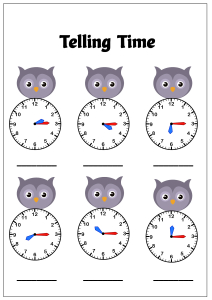# Math Worksheet : Telling Time to the Quarter Hour (Quarter Past) - Owl Theme Time

Practice telling time on analog clocks to the quarter hour (Quarter Past) - Help kids learn telling time to the Quarter Hour using the analog clocks. These worksheets will help kids learn and practice telling and writing the time correct to the quarter hour. Introduce the concept of time using our Tell time to hour or half hour or quarter hour Owl theme worksheets collection.Viewed by 3112 parents and educators
Age Group
Subjects

#### Related Worksheets

Related worksheet for Kids

• Math

• Math

• Math

• Math

• Math

• Math